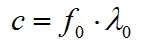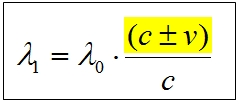MEANING OF WAVELENGTH CHANGE?Han Erim

Jun 2018FACTORY SETTINGS OF A SIGNAL TRANSMITTER:Signal speed = frequency x wavelengthWAVELENGTH CHANGE:We have seen in the book many times that the part of (c + v) in the above Doppler Equation is the speed of the signal according to the reference system of the signal source.From the above two equations, we can obtain following equation:RELATIVE TO SIGNAL SOURCE:SIGNAL SPEED SENT TO THE MOVING TARGETSignal speed = Frequency of the transmitter x Changed wavelengthMEANING OF THE WAVELENGTH CHANGE: